# 前言

• pyhton 2.7.8
• numpy 1.11.1
• scipy 0.16.1
• matplotlib 1.5.1

# 三剑客之Numpy

numpy是一个开源的python科学计算库，包含了很多实用的数学函数，涵盖线性代数、傅里叶变换和随机数生成等功能。最初的numpy其实是scipy的一部分，后来才从scipy中分离出来。

numpy不是python的标准库，需要单独安装。假定你的运行环境已经安装了python包管理工具pip，numpy的安装就非常简单：

pip install numpy


## 数组对象

ndarray是多维数组对象，也是numpy最核心的对象。在numpy中，数组的维度(dimensions)叫做轴(axes)，轴的个数叫做秩(rank)。通常，一个numpy数组的所有元素都是同一种类型的数据，而这些数据的存储和数组的形式无关。

import numpy as np
a = np.array([[1,2,3],[4,5,6],[7,8,9]])


### 数据类型

numpy支持的数据类型主要有布尔型(bool)、整型(integrate)、浮点型(float)和复数型(complex)，每一种数据类型根据占用内存的字节数又分为多个不同的子类型。常见的数据类型见下表。

bool 用1位存储的布尔类型（值为TRUE或FALSE）
inti 由所在平台决定其精度的整数（一般为int32或int64）
int8 1字节整数
int16 2字节整数
int32 4字节整数
int64 8字节整数
uint8 1字节无符号整数
uint16 2字节无符号整数
uint32 4字节无符号整数
uint64 8字节无符号整数
float16 半精度浮点数（16位），1位符号，5位指数，10位尾数
float32 单精度浮点数（32位），1位符号，8位指数，23位尾数
float64/float 双精度浮点数（64位），1位符号，11位指数，52位尾数
complex64 复数，分别用32位表示实部和虚部
complex128/complex 复数，分别用64位表示实部和虚部

### 创建数组

>>> np.array([[1,2,3],[4,5,6]])                # 默认元素类型为int32
array([[1, 2, 3],
[4, 5, 6]])
>>> np.array([[1,2,3],[4,5,6]], dtype=np.int8) # 指定元素类型为int8
array([[1, 2, 3],
[4, 5, 6]], dtype=int8)
>>> np.arange(5)                               # 默认元素类型为int32
array([0, 1, 2, 3, 4])
>>> np.arange(3,8, dtype=np.int8)              # 指定元素类型为int8
array([3, 4, 5, 6, 7], dtype=int8)
>>> np.arange(12).reshape(3,4)                 # 改变shape
array([[ 0,  1,  2,  3],
[ 4,  5,  6,  7],
[ 8,  9, 10, 11]])
>>> np.linspace(1,2,5)                        # 从1到2生成5个浮点数
array([ 1.  ,  1.25,  1.5 ,  1.75,  2.  ])
>>> np.zeros((2,3))                            # 全0数组
array([[ 0.,  0.,  0.],
[ 0.,  0.,  0.]])
>>> np.ones((2,3))                             # 全1数组
array([[ 1.,  1.,  1.],
[ 1.,  1.,  1.]])
>>> np.eye(3)                                  # 主对角线元素为1其他元素为0
array([[ 1.,  0.,  0.],
[ 0.,  1.,  0.],
[ 0.,  0.,  1.]])
>>> np.random.random((2,3))                    # 生成[0,1)之间的随机浮点数
array([[ 0.84731148,  0.8222318 ,  0.85799278],
[ 0.59371558,  0.92330741,  0.04518351]])
>>> np.random.randint(0,10,(3,2))              # 生成[0,10)之间的随机整数
array([[2, 4],
[8, 3],
[8, 5]])


### 构造复杂数组

#### 重复数组: tile

>>> a = np.arange(5)
>>> a
array([0, 1, 2, 3, 4])
>>> np.tile(a, 2)
array([0, 1, 2, 3, 4, 0, 1, 2, 3, 4])
>>> np.tile(a, (3,2))
array([[0, 1, 2, 3, 4, 0, 1, 2, 3, 4],
[0, 1, 2, 3, 4, 0, 1, 2, 3, 4],
[0, 1, 2, 3, 4, 0, 1, 2, 3, 4]])


#### 重复元素: repeat

>>> a = np.arange(5)
>>> a
array([0, 1, 2, 3, 4])
>>> a.repeat(2)
array([0, 0, 1, 1, 2, 2, 3, 3, 4, 4])


#### 一维数组网格化: meshgrid

>>> a = np.arange(5)
>>> b = np.arange(5,10)
>>> np.meshgrid(a,b)
[array([[0, 1, 2, 3, 4],
[0, 1, 2, 3, 4],
[0, 1, 2, 3, 4],
[0, 1, 2, 3, 4],
[0, 1, 2, 3, 4]]), array([[5, 5, 5, 5, 5],
[6, 6, 6, 6, 6],
[7, 7, 7, 7, 7],
[8, 8, 8, 8, 8],
[9, 9, 9, 9, 9]])]
>>>


#### 指定范围和分割方式的网格化: mgrid

>>> np.mgrid[0:1:2j, 1:2:3j]
array([[[ 0. ,  0. ,  0. ],
[ 1. ,  1. ,  1. ]],

[[ 1. ,  1.5,  2. ],
[ 1. ,  1.5,  2. ]]])
>>> np.mgrid[0:1:0.3, 1:2:0.4]
array([[[ 0. ,  0. ,  0. ],
[ 0.3,  0.3,  0.3],
[ 0.6,  0.6,  0.6],
[ 0.9,  0.9,  0.9]],

[[ 1. ,  1.4,  1.8],
[ 1. ,  1.4,  1.8],
[ 1. ,  1.4,  1.8],
[ 1. ,  1.4,  1.8]]])


>>> complex(2,5)
(2+5j)


### 数组的属性

numpy的数组对象除了一些常规的属性外，也有几个类似转置、扁平迭代器等看起来更像是方法的属性。扁平迭代器也许是遍历多维数组的一个简明方法，下面的代码给出了一个例子。

>>> a = np.array([[1,2,3],[4,5,6]])
>>> a.dtype               # 数组元素的数据类型
dtype('int32')
>>> a.dtype.itemsize      # 数组元素占据的内存字节数
4
>>> a.itemsize            # 数组元素占据的内存字节数
4
>>> a.shape               # 数组的维度
(2, 3)
>>> a.size                # 数组元素个数
6
>>> a.T                   # 数组行变列，类似于transpose()
array([[1, 4],
[2, 5],
[3, 6]])
>>> a.flat                # 返回一个扁平迭代器，用于遍历多维数组
<numpy.flatiter object at 0x037188F0>
>>> for item in a.flat:
print item
1
2
...


### 改变数组维度

numpy数组的存储顺序和数组的维度是不相干的，因此改变数组的维度是非常便捷的操作，除resize()外，这一类操作不会改变所操作的数组本身的存储顺序。

>>> a = np.array([[1,2,3],[4,5,6]])
>>> a.shape                     # 查看数组维度
(2, 3)
>>> a.reshape(3,2)              # 返回3行2列的数组
array([[1, 2],
[3, 4],
[5, 6]])
>>> a.ravel()                   # 返回一维数组
array([1, 2, 3, 4, 5, 6])
>>> a.transpose()               # 行变列（类似于矩阵转置）
array([[1, 4],
[2, 5],
[3, 6]])
>>> a.resize((3,2))             # 类似于reshape，但会改变所操作的数组
>>> a
array([[1, 2],
[3, 4],
[5, 6]])


### 索引和切片

a = np.arange(9)
>>> a[-1]                            # 最后一个元素
8
>>> a[2:5]                           # 返回第2到第5个元素
array([2, 3, 4])
>>> a[:7:3]                          # 返回第0到第7个元素，步长为3
array([0, 3, 6])
>>> a[::-1]                          # 返回逆序的数组
array([8, 7, 6, 5, 4, 3, 2, 1, 0])


>>> a = np.arange(24).reshape(2,3,4)    # 2层3排4列
>>> a
array([[[ 0,  1,  2,  3],
[ 4,  5,  6,  7],
[ 8,  9, 10, 11]],

[[12, 13, 14, 15],
[16, 17, 18, 19],
[20, 21, 22, 23]]])
>>> a                          # 虽然可以这样
23
>>> a[1,2,3]                            # 但这才是规范的用法
23
>>> a[:,0,0]                            # 所有楼层的第1排第1列
array([ 0, 12])
>>> a[0,:,:]                            # 1楼的所有房间，等价与a或a[0,...]
array([[ 0,  1,  2,  3],
[ 4,  5,  6,  7],
[ 8,  9, 10, 11]])
>>> a[:,:,1:3]                          # 所有楼层所有排的第2到4列
array([[[ 1,  2],
[ 5,  6],
[ 9, 10]],

[[13, 14],
[17, 18],
[21, 22]]])
>>> a[1,:,-1]                           # 2层每一排的最后一个房间
array([15, 19, 23])


### 数组合并

>>> a = np.arange(9).reshape(3,3)
>>> b = np.arange(9,18).reshape(3,3)
>>> a
array([[0, 1, 2],
[3, 4, 5],
[6, 7, 8]])
>>> b
array([[ 9, 10, 11],
[12, 13, 14],
[15, 16, 17]])
>>> np.hstack((a,b))                        # 水平合并
array([[ 0,  1,  2,  9, 10, 11],
[ 3,  4,  5, 12, 13, 14],
[ 6,  7,  8, 15, 16, 17]])
>>> np.vstack((a,b))                        # 垂直合并
array([[ 0,  1,  2],
[ 3,  4,  5],
[ 6,  7,  8],
[ 9, 10, 11],
[12, 13, 14],
[15, 16, 17]])
>>> np.dstack((a,b))                        # 深度合并
array([[[ 0,  9],
[ 1, 10],
[ 2, 11]],

[[ 3, 12],
[ 4, 13],
[ 5, 14]],

[[ 6, 15],
[ 7, 16],
[ 8, 17]]])


### 数组拆分

>>> a = np.arange(9).reshape(3,3)
>>> np.hsplit(a, 3)                        # 水平拆分，返回list
[array([,
,
]), array([,
,
]), array([,
,
])]
>>> np.vsplit(a, 3)                        # 垂直拆分，返回list
[array([[0, 1, 2]]), array([[3, 4, 5]]), array([[6, 7, 8]])]
>>> a = np.arange(27).reshape(3,3,3)
>>> np.dsplit(a, 3)                        # 深度拆分，返回list
[array([[[ 0],
[ 3],
[ 6]],

[[ 9],
,
],

[,
,
]]), array([[[ 1],
[ 4],
[ 7]],

[,
,
],

[,
,
]]), array([[[ 2],
[ 5],
[ 8]],

[,
,
],

[,
,
]])]


### 数组运算

>>> a = np.arange(4, dtype=np.float32).reshape(2,2)
>>> b = np.arange(4, 8, dtype=np.float32).reshape(2,2)
>>> a+2                # 数组和常数可以进行四则运算
array([[ 2.,  3.],
[ 4.,  5.]], dtype=float32)
>>> a/b                # 数组和数组可以进行四则运算
array([[ 0.        ,  0.2       ],
[ 0.33333334,  0.42857143]], dtype=float32)
>>> a == b             # 最神奇的是，数组可以判断对应元素是否相等
array([[False, False],
[False, False]], dtype=bool)
>>> (a == b).all()     # 判断数组是否相等
False


>>> a = np.arange(6).reshape((2,3))
>>> a
array([[0, 1, 2],
[3, 4, 5]])
>>> (a>2)&(a<=4)
array([[False, False, False],
[ True,  True, False]], dtype=bool)
>>> a[(a>2)&(a<=4)]
array([3, 4])
>>> a[(a>2)&((a<=4))] += 10
>>> a
array([[ 0,  1,  2],
[13, 14,  5]])


### 数组方法和常用函数

>>> a = np.array([3,2,4])
>>> a.sum()                   # 所有元素的和
9
>>> a.prod()                  # 所有元素的乘积
24
>>> a.mean()                  # 所有元素的算数平均值
3.0
>>> a.max()                   # 所有元素的最大值
4
>>> a.min()                   # 所有元素的最小值
2
>>> a.clip(3,4)               # 小于3的元素替换为3，大于4的元素替换为4
array([3, 3, 4])
>>> a.compress(a>2)           # 返回大于2的元素组成的数组
array([3, 4])
>>> a.tolist()                # 返回python的list
[3, 2, 4]
>>> a.var()                   # 计算方差（元素与均值之差的平方的均值）
0.66666666666666663
>>> a.std()                   # 计算标准差（方差的算术平方根）
0.81649658092772603
>>> a.ptp()                   # 返回数组的最大值和最小值之差
2
>>> a.argmin()                # 返回最小值在扁平数组中的索引
1
>>> a.argmax()                # 返回最大值在扁平数组中的索引
2
>>> np.where(a == 2)          # 返回所有值为2的元素的索引
(array(),)
>>> np.diff(a)                # 返回相邻元素的差
array([-1,  2])
>>> np.log(a)                 # 返回对数数组
array([ 1.09861229,  0.69314718,  1.38629436])
>>> np.exp(a)                 # 返回指数数组
array([ 20.08553692,   7.3890561 ,  54.59815003])
>>> np.sqrt(a)                # 返回开方数组
array([ 1.73205081,  1.41421356,  2.        ])
>>> np.msort(a)               # 数组排序
array([2, 3, 4])
>>> a = np.array([1,4,7])
>>> b = np.array([8,5,2])
>>> np.maximum(a, b)          # 返回多个数组中对应位置元素的最大值数组
array([8, 5, 7])
>>> np.minimum(a, b)          # 返回多个数组中对应位置元素的最小值数组
array([1, 4, 2])
>>> np.true_divide(a, b)      # 对整数实现真正的数学除法运算
array([ 0.125,  0.8  ,  3.5  ])


## 矩阵对象

matrix是矩阵对象，继承自ndarray类型，因此含有ndarray的所有数据属性和方法。不过，当你把矩阵对象当数组操作时，需要注意以下几点：

• matrix对象总是二维的，即使是展平（ravel函数）操作或是成员选择，返回值也是二维的
• matrix对象和ndarray对象混合的运算总是返回matrix对象

### 创建矩阵

matrix对象可以使用一个Matlab风格的字符串来创建（以空格分隔列，以分号分隔行的字符串），也可以用数组来创建。

>>> np.mat('1 4 7; 2 5 8; 3 6 9')
matrix([[1, 4, 7],
[2, 5, 8],
[3, 6, 9]])
>>> np.mat(np.arange(1,10).reshape(3,3))
matrix([[1, 2, 3],
[4, 5, 6],
[7, 8, 9]])


### 矩阵的特有属性

>>> m = np.mat(np.arange(1,10).reshape(3,3))
>>> m
matrix([[1, 2, 3],
[4, 5, 6],
[7, 8, 9]])
>>> m.T             # 返回自身的转置
matrix([[1, 4, 7],
[2, 5, 8],
[3, 6, 9]])
>>> m.H             # 返回自身的共轭转置
matrix([[1, 4, 7],
[2, 5, 8],
[3, 6, 9]])
>>> m.I             # 返回自身的逆矩阵
matrix([[ -4.50359963e+15,   9.00719925e+15,  -4.50359963e+15],
[  9.00719925e+15,  -1.80143985e+16,   9.00719925e+15],
[ -4.50359963e+15,   9.00719925e+15,  -4.50359963e+15]])
>>> m.A             # 返回自身数据的二维数组的一个视图
array([[1, 2, 3],
[4, 5, 6],
[7, 8, 9]])


### 矩阵乘法

>>> a = np.array([1,2,3])
>>> b = np.array([4,5,6])
>>> a*b               # 一维数组，元素相乘
array([ 4, 10, 18])
>>> np.dot(a,b)       # 一维数组，元素相乘再求和
32
>>> a = np.array([[1,2],[3,4]])
>>> b = np.array([[5,6],[7,8]])
>>> a*b               # 多维数组，元素相乘
array([[ 5, 12],
[21, 32]])
>>> np.dot(a,b)       # 多维数组，实现的是矩阵相乘
array([[19, 22],
[43, 50]])
>>> m = np.mat(a)
>>> n = np.mat(b)
>>> np.dot(m,n)       # 矩阵相乘
matrix([[19, 22],
[43, 50]])
>>> m*n               # 矩阵相乘
matrix([[19, 22],
[43, 50]])


## 线性代数模块

numpy.linalg 是numpy的线性代数模块，可以用来解决逆矩阵、特征值、线性方程组以及行列式等问题。

### 计算逆矩阵

m = np.mat('0 1 2; 1 0 3; 4 -3 8')
mi = np.linalg.inv(m)           # mi即为m的逆矩阵。何以证明？
m * mi                          # 矩阵与其逆矩阵相乘，结果为单位矩阵
matrix([[ 1.,  0.,  0.],
[ 0.,  1.,  0.],
[ 0.,  0.,  1.]])


### 计算行列式

m = np.mat('0 1 2; 1 0 3; 4 -3 8')
np.linalg.det(m)                # 什么？这就成了？
2.0


### 计算特征值和特征向量

m = np.mat('0 1 2; 1 0 3; 4 -3 8')
>>> np.linalg.eigvals(m)        # 计算特征值
array([ 7.96850246, -0.48548592,  0.51698346])
>>> np.linalg.eig(m)            # 返回特征值及其对应特征向量的元组
(array([ 7.96850246, -0.48548592,  0.51698346]), matrix([[ 0.26955165,  0.90772191, -0.74373492],
[ 0.36874217,  0.24316331, -0.65468206],
[ 0.88959042, -0.34192476,  0.13509171]]))


### 求解线性方程组

x - 2y + z = 0
2y -8z = 8
-4x + 5y + 9z = -9

>>> A = np.mat('1 -2 1; 0 2 -8; -4 5 9')
>>> b = np.array([0, 8, -9])
>>> np.linalg.solve(A, b)
array([ 29.,  16.,   3.])  # x = 29, y = 16, z = 3


# 三剑客之Matplotlib

matplotlib 是python最著名的绘图库，它提供了一整套和Matlab相似的命令API，十分适合交互式地进行制图。而且也可以方便地将它作为绘图控件，嵌入GUI应用程序中。matplotlib 可以绘制多种形式的图形包括普通的线图，直方图，饼图，散点图以及误差线图等；可以比较方便的定制图形的各种属性比如图线的类型，颜色，粗细，字体的大小等；它能够很好地支持一部分 TeX 排版命令，可以比较美观地显示图形中的数学公式。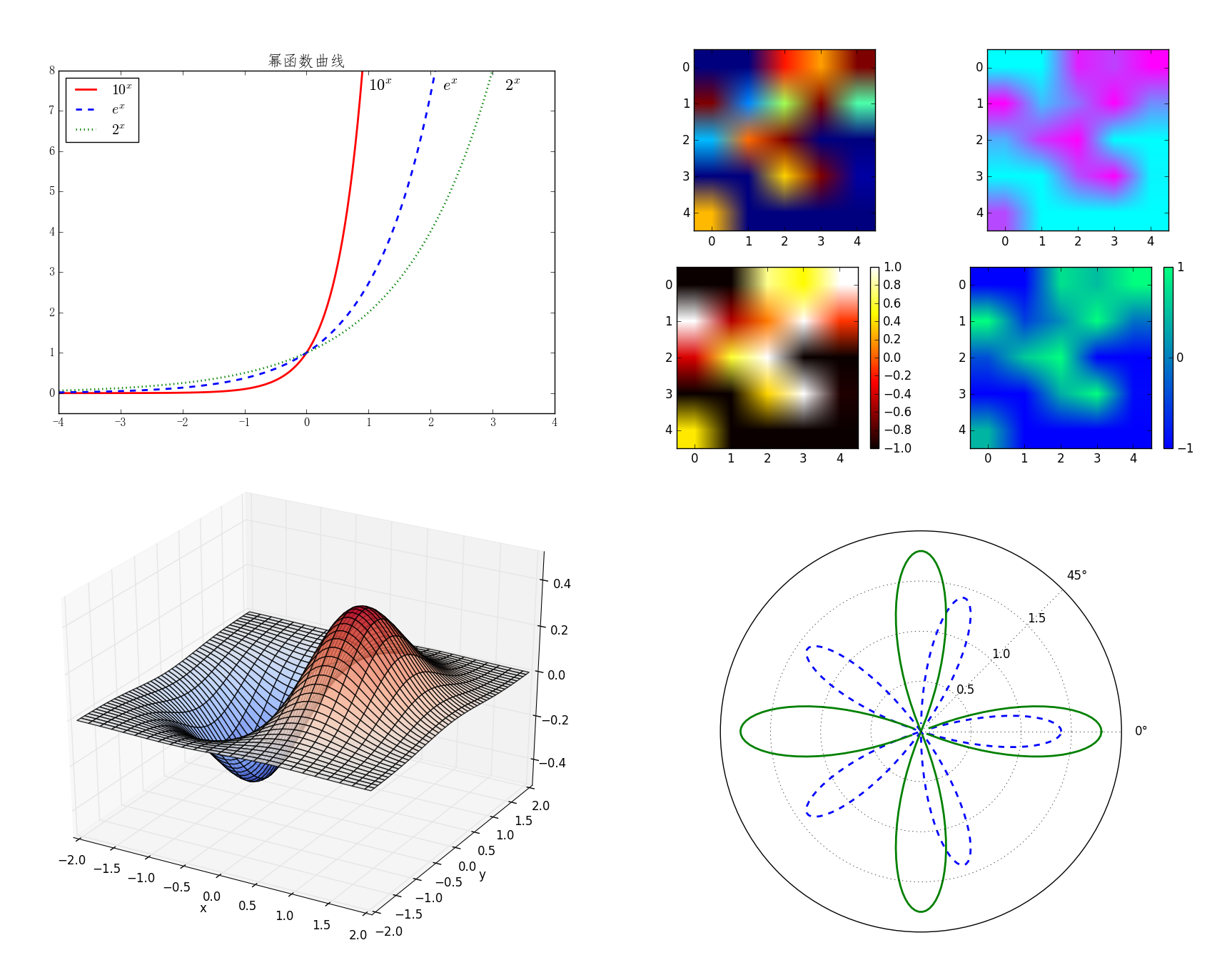## pylot介绍

Matplotlib 包含了几十个不同的模块， 如 matlab、mathtext、finance、dates 等，而 pylot 则是我们最常用的绘图模块，这也是本文介绍的重点。

### 中文显示问题的解决方案

>>> from pylab import mpl
>>> mpl.rcParams['font.sans-serif'] = ['FangSong'] # 指定默认字体
>>> mpl.rcParams['axes.unicode_minus'] = False     # 解决保存图像时'-'显示为方块的问题


### 绘制最简单的图形

>>> import numpy as np
>>> import matplotlib.pyplot as plt
>>> x = np.arange(0, 2*np.pi, 0.01)
>>> y = np.sin(x)
>>> plt.plot(x, y)
>>> plt.show()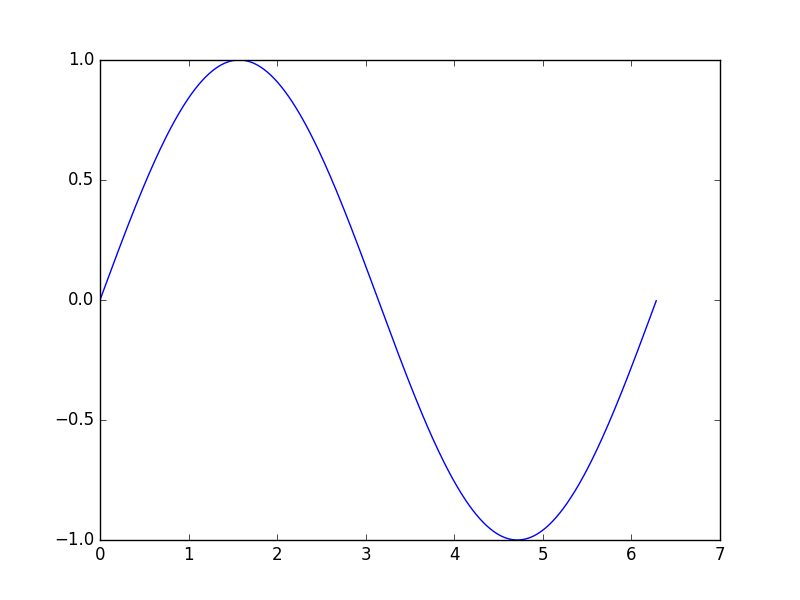### 设置标题、坐标轴名称、坐标轴范围

>>> import numpy as np
>>> import matplotlib.pyplot as plt
>>> from pylab import mpl
>>> mpl.rcParams['font.sans-serif'] = ['FangSong']
>>> mpl.rcParams['axes.unicode_minus'] = False
>>> x = np.arange(0, 2*np.pi, 0.01)
>>> y = np.sin(x)
>>> plt.plot(x, y)
>>> plt.title(u'正弦曲线', fontdict={'size':20})      # 设置标题
>>> plt.xlabel(u'弧度', fontdict={'size':16})         # 显示横轴名称
>>> plt.ylabel(u'正弦值', fontdict={'size':16})       # 显示纵轴名称
>>> plt.axis([-0.1*np.pi, 2.1*np.pi, -1.1, 1.1])     # 设置坐标轴范围
>>> plt.show()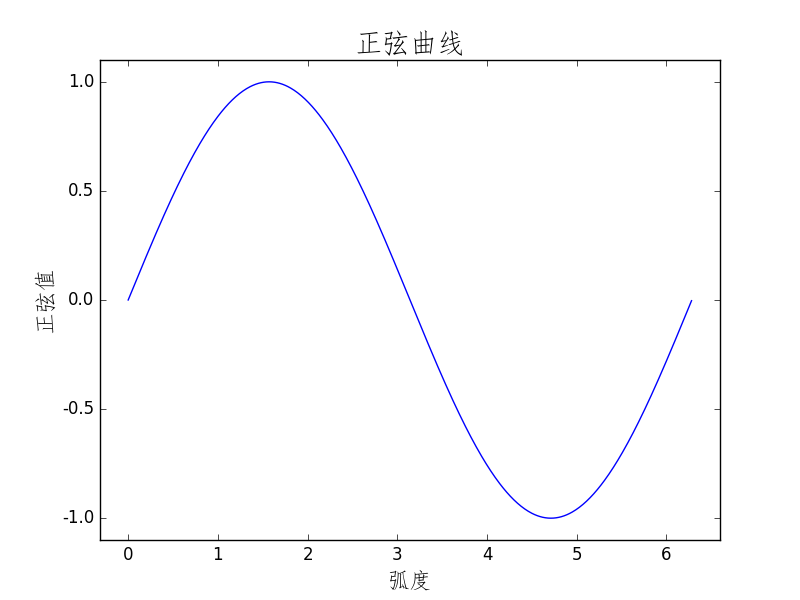### 设置点和线的样式、宽度、颜色

plt.plot函数的调用形式如下：

plot(x, y, color='green', linestyle='dashed', linewidth=1, marker='o', markerfacecolor='blue', markersize=6)
plot(x, y, c='g', ls='--', lw=1, marker='o', mfc='blue', ms=6)

1. color指定线的颜色，可简写为“c”。颜色的选项为：
• 蓝色： ‘b’ (blue)
• 绿色： ‘g’ (green)
• 红色： ‘r’ (red)
• 墨绿： ‘c’ (cyan)
• 洋红： ‘m’ (magenta)
• 黄色： ‘y’ (yellow)
• 黑色： ‘k’ (black)
• 白色： ‘w’ (white)
• 灰度表示： e.g. 0.75 ([0,1]内任意浮点数)
• RGB表示法： e.g. ‘#2F4F4F’ 或 (0.18, 0.31, 0.31)
1. linestyle指定线型，可简写为“ls”。线型的选项为：
• 实线： ‘-’ (solid line)
• 虚线： ‘–’ (dashed line)
• 虚点线： ‘-.’ (dash-dot line)
• 点线： ‘:’ (dotted line)
• 无： '‘或’ ‘或’None’
1. linewidth指定线宽，可简写为“lw”。

2. marker描述数据点的形状

• 点线： ‘.’
• 点线： ‘o’
• 加号： '+
• 叉号： ‘x’
• 上三角： ‘^’
• 上三角： ‘v’
1. markerfacecolor指定数据点标记的表面颜色，可 简写为“ mfc”。

2. markersize指定数据点标记的大小，可 简写为“ ms”。

### 文本标注和图例

>>> import numpy as np
>>> import matplotlib.pyplot as plt
>>> from pylab import mpl
>>> mpl.rcParams['font.sans-serif'] = ['FangSong']
>>> mpl.rcParams['axes.unicode_minus'] = False
>>> x = np.linspace(-4, 4, 200)
>>> f1 = np.power(10, x)
>>> f2 = np.power(np.e, x)
>>> f3 = np.power(2, x)
>>> plt.plot(x, f1, 'r', ls='-', linewidth=2, label='$10^x$')
>>> plt.plot(x, f2, 'b', ls='--', linewidth=2, label='$e^x$')
>>> plt.plot(x, f3, 'g', ls=':', linewidth=2, label='$2^x$')
>>> plt.axis([-4, 4, -0.5, 8])
>>> plt.text(1, 7.5, r'$10^x$', fontsize=16)
>>> plt.text(2.2, 7.5, r'$e^x$', fontsize=16)
>>> plt.text(3.2, 7.5, r'$2^x$', fontsize=16)
>>> plt.title('幂函数曲线', fontsize=16)
>>> plt.legend(loc='upper left')
>>> plt.show()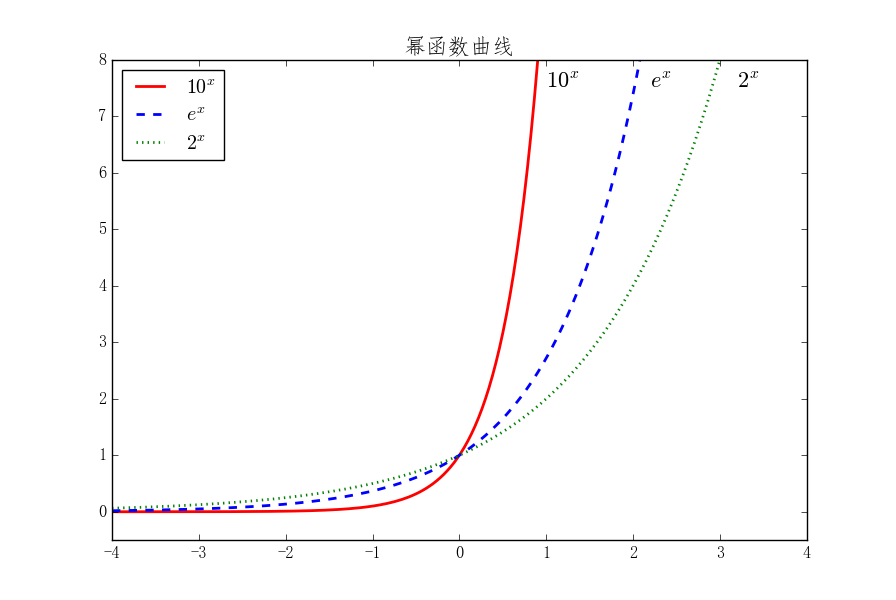• best
• upper right
• upper left
• lower left
• lower right

## 绘制多轴图

subplot(numRows, numCols, plotNum)


subplot将整个绘图区域等分为numRows行 * numCols列个子区域，然后按照从左到右，从上到下的顺序对每个子区域进行编号，左上的子区域的编号为1。如果numRows，numCols和plotNum这三个数都小于10的话，可以把它们缩写为一个整数，例如subplot(323)和subplot(3,2,3)是相同的。subplot在plotNum指定的区域中创建一个轴对象。如果新创建的轴和之前创建的轴重叠的话，之前的轴将被删除。

>>> import matplotlib.pyplot as plt
>>> plt.subplot(221) # 两行两列的第1个位置
>>> plt.axis([-1, 2, -1, 2])
>>> plt.axhline(y=0.5, color='b')
>>> plt.axhline(y=0.5, xmin=0.25, xmax=0.75, color='r')
>>> plt.subplot(222) # 两行两列的第2个位置
>>> plt.axis([-1, 2, -1, 2])
>>> plt.axvline(x=0, ymin=0, linewidth=4, color='r')
>>> plt.axvline(x=1.0, ymin=-0.5, ymax=0.5, linewidth=4, color='g')
>>> plt.subplot(212) # 两行一列的第2个位置
>>> plt.axis([-1, 2, -1, 2])
>>> plt.axvspan(1.25, 1.55, facecolor='g', alpha=0.5)
>>> plt.axhspan(0.25, 0.75, facecolor='0.5', alpha=0.5)
>>> plt.show()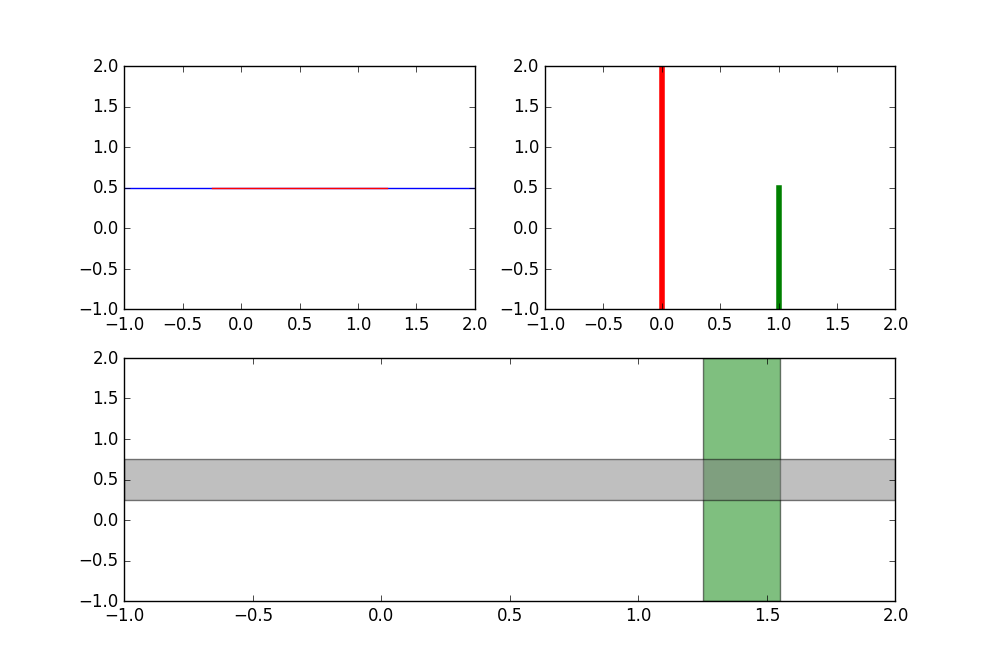## 常用绘图类型

### 直方图

>>> import numpy as np
>>> import matplotlib.pyplot as plt
>>> data = np.random.normal(5.0, 3.0, 1000)
>>> plt.hist(data)
>>> bins = np.arange(-5, 16, 1)
>>> plt.hist(data, bins) # 使用自定义的分段区域
>>> plt.show()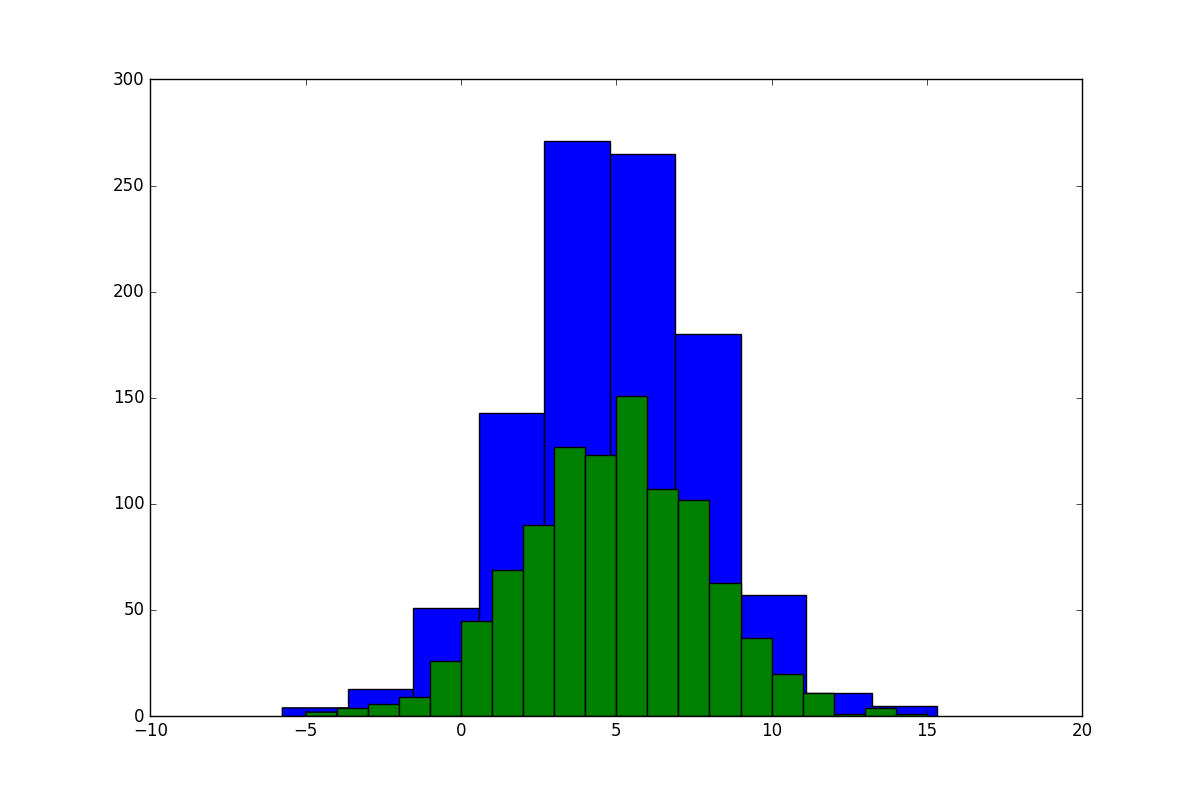### 散点图

plt.scatter函数的调用形式如下：

scatter(x, y, s=None, c=None, marker=None, cmap=None, norm=None, vmin=None, vmax=None, alpha=None, linewidths=None, verts=None, edgecolors=None, hold=None, data=None, **kwargs)


scatter()的前两个参数是数组，分别指定每个点的X轴和Y轴的坐标。s参数指定点的大 小，值和点的面积成正比，它可以是一个数，指定所有点的大小，也可以是数组，分别对每个点指定大小。c参数指定每个点的颜色，可以是数值或数组。这里使用一维数组为每个点指定了一个数值。通过颜色映射表，每个数值都会与一个颜色相对应。默认的颜色映射表中蓝色与最小值对应，红色与最大值对应。当c参数是形状为(N,3)或(N,4)的二维数组时，则直接表示每个点的RGB颜色。marker参数设置点的形状，可以是个表示形状的字符串，也可以是表示多边形的两个元素的元组，第一个元素表示多边形的边数，第二个元素表示多边形的样式，取值范围为0、1、2、3。0表示多边形，1表示星形，2表示放射形，3表示忽略边数而显示为圆形。alpha参数设置点的透明度。facecolors参数为“none”时，表示散列点没有填充色。

>>> import numpy as np
>>> import matplotlib.pyplot as plt
>>> x = np.random.rand(50)
>>> y = np.random.rand(50)
>>> area = np.pi * (15 * np.random.rand(50))**2
>>> color = 2 * np.pi * np.random.rand(50)
>>> plt.scatter(x, y, s=area, c=color, alpha=0.5, cmap=plt.cm.hsv)
>>> plt.show()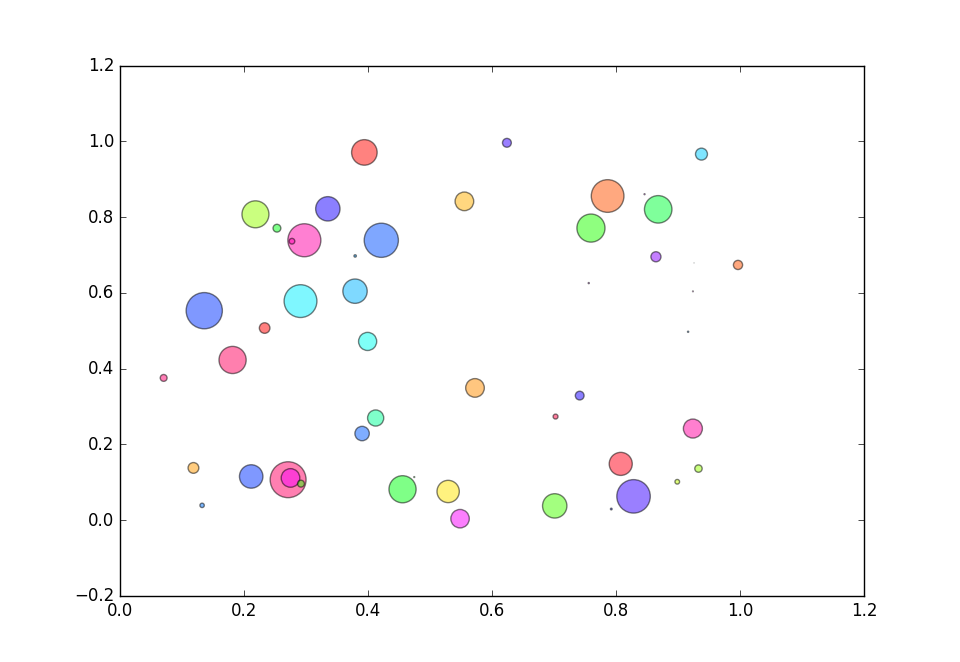### 梯形图、柱状图、填充图

>>> import numpy as np
>>> import matplotlib.pyplot as plt
>>> n = np.array([0,1,2,3,4,5])
>>> x = np.linspace(-0.75, 1., 100)
>>> plt.subplot(131)
>>> plt.step(n, n**2, lw=2)
>>> plt.subplot(132)
>>> plt.bar(n, n**2, align="center", width=0.5, alpha=0.5)
>>> plt.subplot(133)
>>> plt.fill_between(x, x**2, x**3, color="green", alpha=0.5)
>>> plt.show()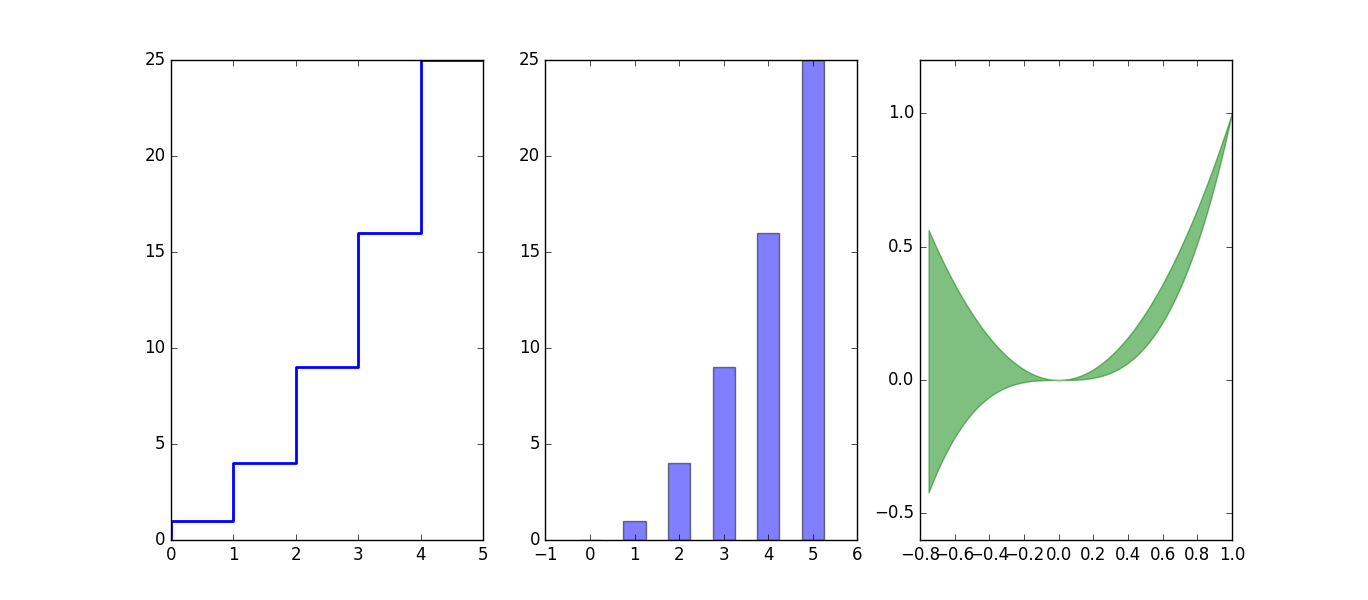### 对数坐标

plot()所绘制图表的X-Y轴坐标都是算术坐标。绘制对数坐标图的函数有三个：semilogx()、semilogy()和loglog(),它们分别绘制X轴为对数坐标、Y轴为对数坐标以及两个轴都为对数坐标时的图表。

>>> import numpy as np
>>> import matplotlib.pyplot as plt
>>> x = np.linspace(0, 3, 100)
>>> y = np.power(2, np.power(2,x))
>>> plt.subplot(121)
>>> plt.semilogy(x, y , '-r')
>>> plt.subplot(122)
>>> plt.plot(x,y, '--g')
>>> plt.show()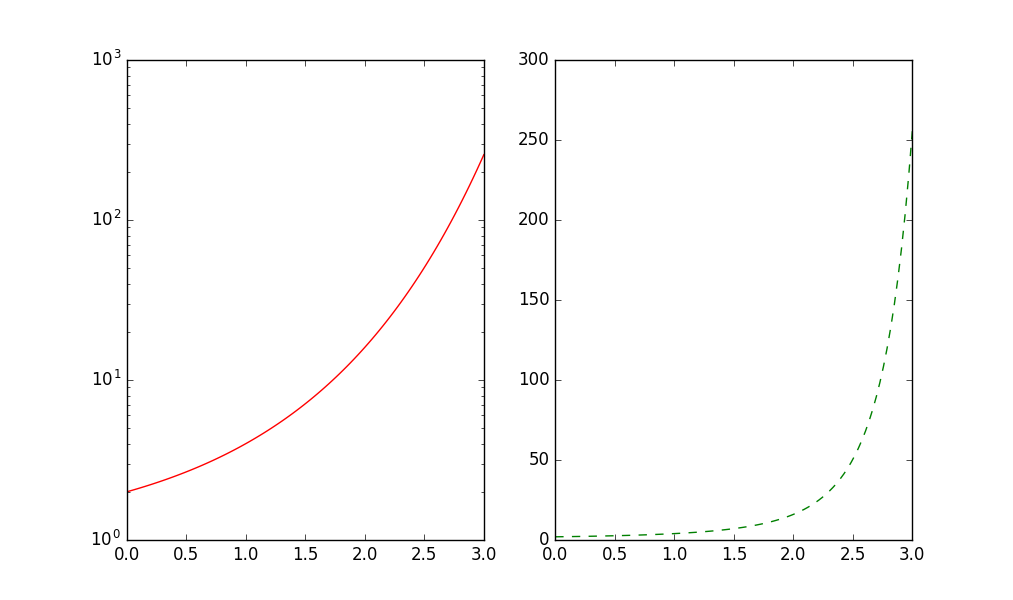### 极坐标绘图

>>> import numpy as np
>>> import matplotlib.pyplot as plt
>>> theta = np.arange(0, 2*np.pi, 0.02)
>>> plt.polar(theta, 1.4*np.cos(5*theta), "--", linewidth=2)
>>> plt.polar(theta, 1.8*np.cos(4*theta), linewidth=2)
>>> plt.rgrids(np.arange(0.5, 2, 0.5), angle=45)
>>> plt.thetagrids([0, 45])thetagridlabel objects>)
>>> plt.show()
>>>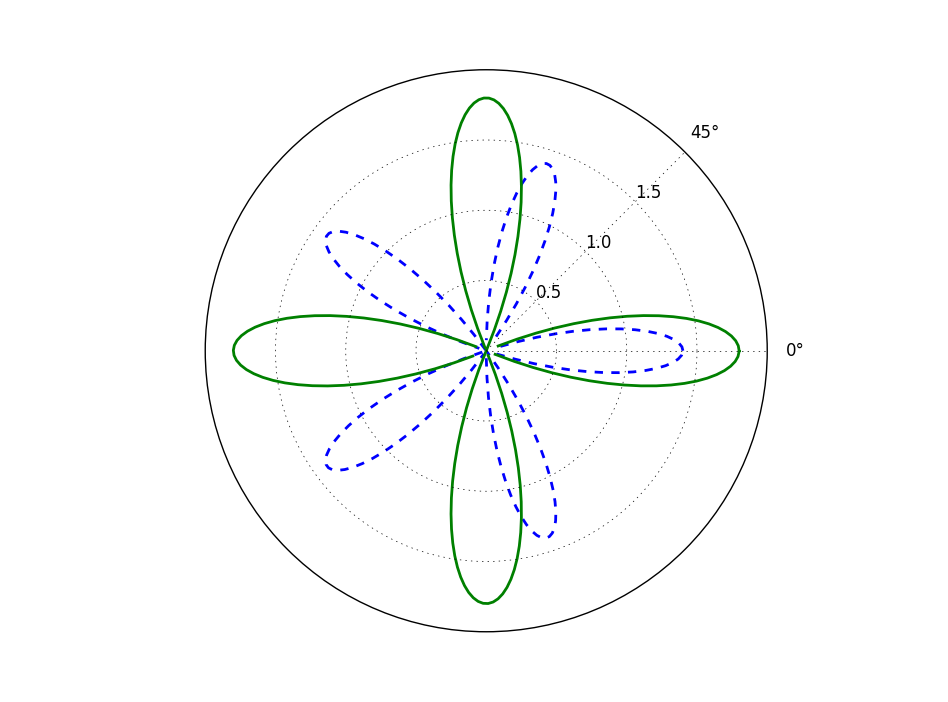## 2D绘图

### 等值线图

>>> import numpy as np
>>> import matplotlib.pyplot as plt
>>> y, x = np.ogrid[-2:2:200j, -3:3:300j]
>>> z = x * np.exp( - x**2 - y**2)
>>> extent = [np.min(x), np.max(x), np.min(y), np.max(y)]
>>> plt.subplot(121)
>>> cs = plt.contour(z, 10, extent=extent)
>>> plt.clabel(cs)
<a list of 8 text.Text objects>
>>> plt.subplot(122)
>>> plt.contourf(x.reshape(-1), y.reshape(-1), z, 20)
>>> plt.show()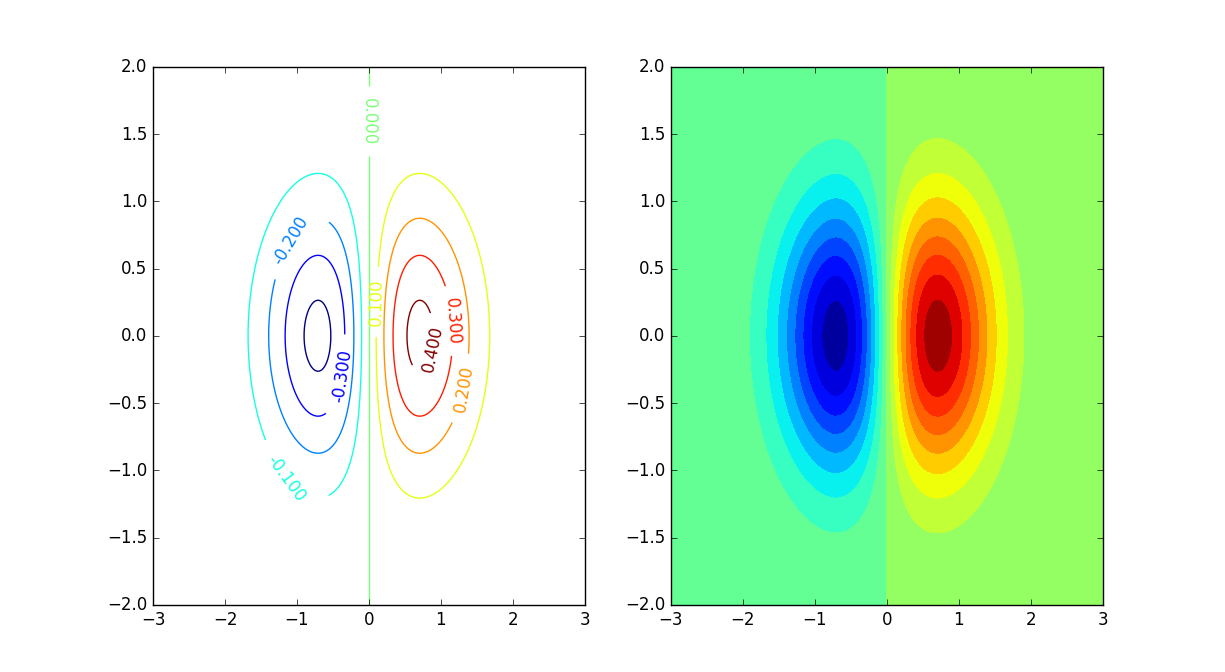### 二维数据的平面色彩显示

>>> import numpy as np
>>> import matplotlib.pyplot as plt
>>> data=np.clip(np.random.randn(5,5),-1,1)
>>> plt.subplot(221)
>>> plt.imshow(data)
>>> plt.subplot(222)
>>> plt.imshow(data,cmap=plt.cm.cool)
>>> plt.subplot(223)
>>> plt.imshow(data,cmap=plt.cm.hot)
>>> plt.colorbar()
>>> plt.subplot(224)
>>> im = plt.imshow(data,cmap=plt.cm.winter)
>>> plt.colorbar(im, cmap=plt.cm.winter, ticks=[-1,0,1])
>>> plt.show()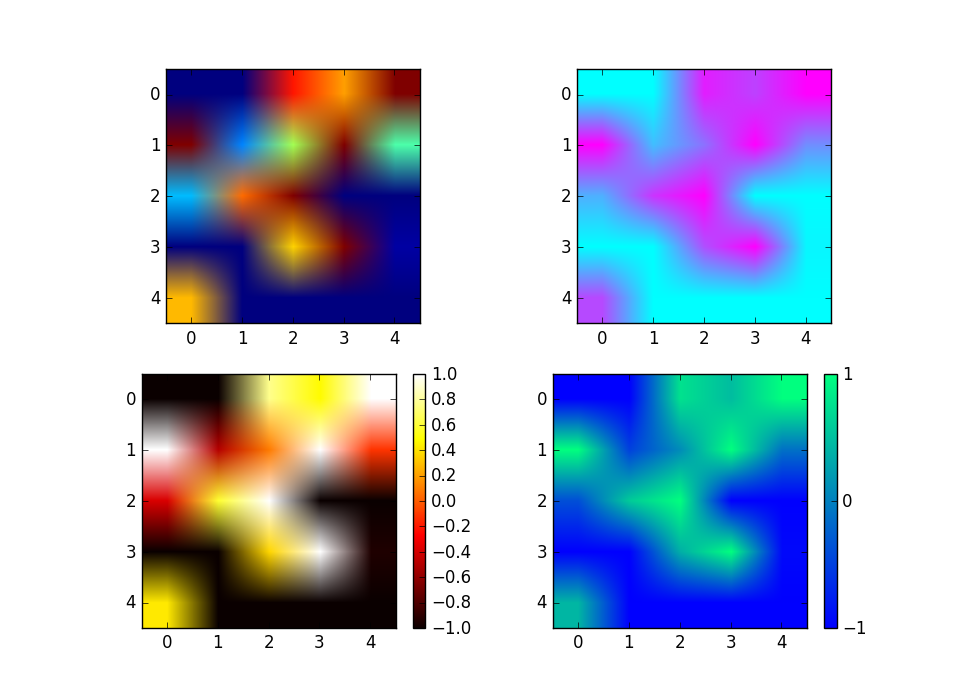## 3D绘图

mpl_toolkits.mplot3工具包提供了一些基本的3D绘图功能，其支持的图表类型包括散点图（scatter）、曲面图（surf）、线图（line）和网格图（mesh）。虽然mplot3d不是一个最好的3D图形绘制库，但是它是伴随着matplotlib产生的，因此我们对其接口已经很熟悉了。

>>> import numpy as np
>>> import matplotlib.pyplot as plt
>>> import mpl_toolkits.mplot3d
>>> x, y = np.mgrid[-2:2:50j,-2:2:50j]
>>> z = x*np.exp(-x**2-y**2)
>>> ax = plt.subplot(111,projection='3d')
>>> ax.plot_surface(x,y,z,rstride=2,cstride=1,cmap=plt.cm.coolwarm,alpha=0.8)
>>> ax.set_xlabel('x')
>>> ax.set_ylabel('y')
>>> ax.set_zlabel('z')
>>> plt.show()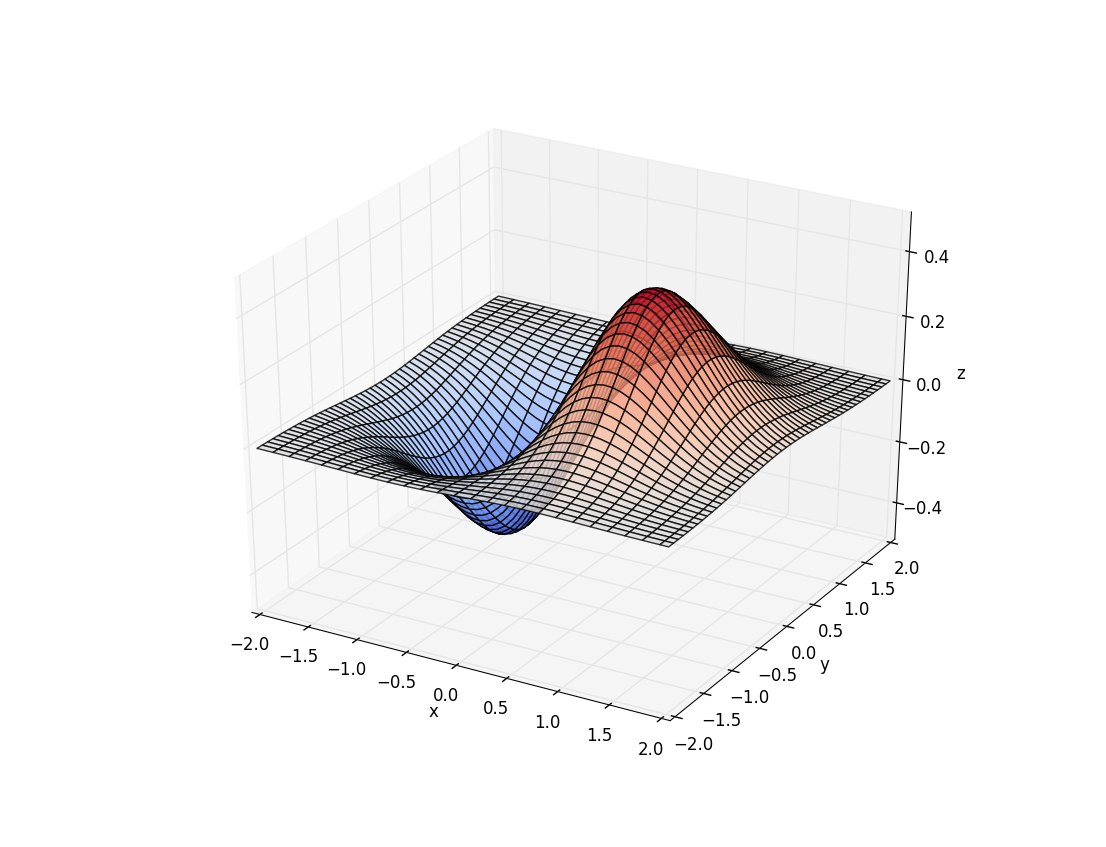# 三剑客之Scipy

## 插值

### 一维插值和样条插值

>>> import numpy as np
>>> from scipy import interpolate
>>> x = np.arange(0,10)
>>> y = np.exp(-x/3.0)
>>> x
array([0, 1, 2, 3, 4, 5, 6, 7, 8, 9])
>>> y
array([ 1.        ,  0.71653131,  0.51341712,  0.36787944,  0.26359714,
0.1888756 ,  0.13533528,  0.09697197,  0.06948345,  0.04978707])
>>> f_line = interpolate.interp1d(x, y)
>>> x_new = np.arange(0,9,0.2)
>>> f_line(x_new)
array([ 1.        ,  0.94330626,  0.88661252,  0.82991879,  0.77322505,
0.71653131,  0.67590847,  0.63528563,  0.5946628 ,  0.55403996,
0.51341712,  0.48430958,  0.45520205,  0.42609451,  0.39698698,
0.36787944,  0.34702298,  0.32616652,  0.30531006,  0.2844536 ,
0.26359714,  0.24865283,  0.23370852,  0.21876422,  0.20381991,
0.1888756 ,  0.17816754,  0.16745947,  0.15675141,  0.14604335,
0.13533528,  0.12766262,  0.11998996,  0.11231729,  0.10464463,
0.09697197,  0.09147426,  0.08597656,  0.08047886,  0.07498115,
0.06948345,  0.06554417,  0.0616049 ,  0.05766562,  0.05372634])
>>> bs = interpolate.splrep(x, y)
>>> interpolate.splev(x_new, bs)
array([ 1.        ,  0.93571489,  0.8754193 ,  0.8189194 ,  0.76602135,
0.71653131,  0.67025545,  0.62699994,  0.58657094,  0.54877461,
0.51341712,  0.48031625,  0.44933621,  0.42035284,  0.39324198,
0.36787944,  0.34414628,  0.32194438,  0.30118082,  0.28176271,
0.26359714,  0.24659576,  0.23068846,  0.2158097 ,  0.20189393,
0.1888756 ,  0.17669225,  0.16529366,  0.15463272,  0.1446623 ,
0.13533528,  0.1266067 ,  0.11844022,  0.11080172,  0.10365702,
0.09697197,  0.09071432,  0.0848594 ,  0.07938446,  0.07426673,
0.06948345,  0.06501185,  0.06082918,  0.05691267,  0.05323955])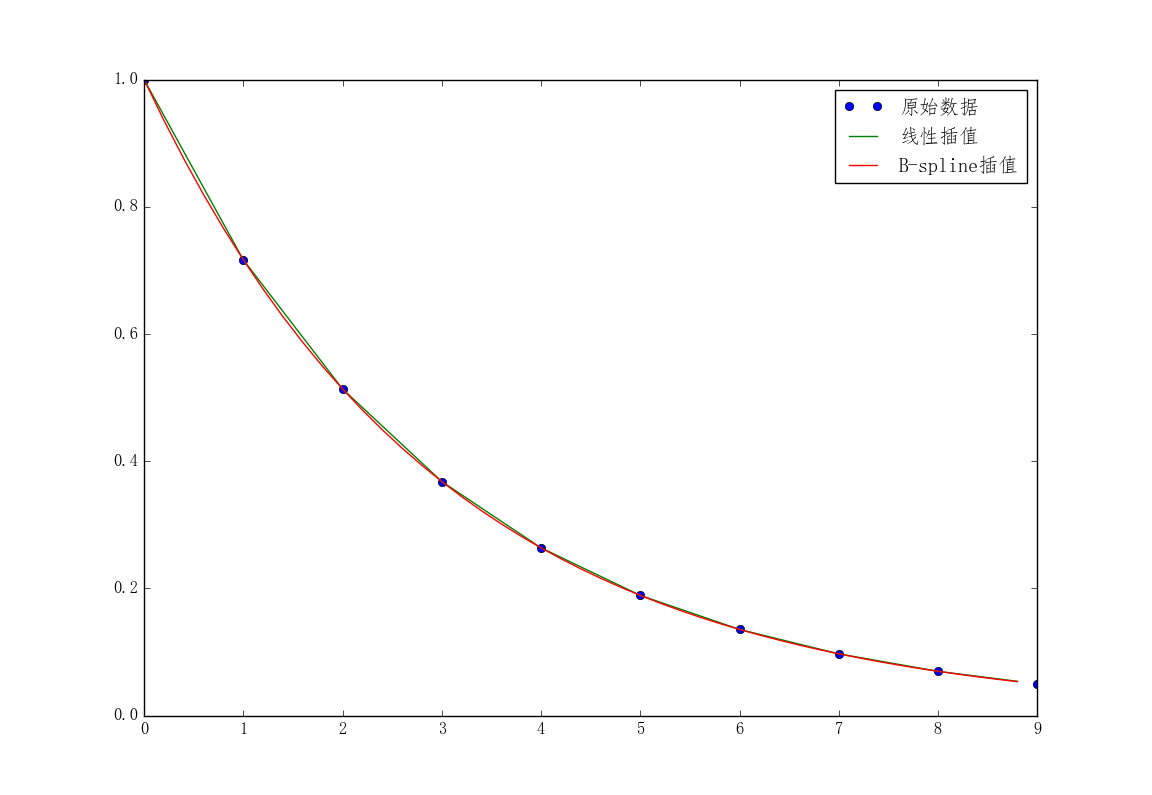import numpy as np
from scipy import interpolate
import matplotlib.pyplot as plt

from pylab import mpl
mpl.rcParams['font.sans-serif'] = ['FangSong'] # 指定默认字体
mpl.rcParams['axes.unicode_minus'] = False     # 解决保存图像时'-'显示为方块的问题

x = np.arange(0,10)
y = np.exp(-x/3.0)

x_new = np.arange(0,9,0.2)
f_linear = interpolate.interp1d(x, y)
bs = interpolate.splrep(x, y)

plt.plot(x, y, "o",  label=u"原始数据")
plt.plot(x_new, f_linear(x_new), label=u"线性插值")
plt.plot(x_new, interpolate.splev(x_new, bs), label=u"B-spline插值")
plt.legend()
plt.show()


scipy.interpolate.splrep(x, y, w=None, xb=None, xe=None, k=3, task=0, s=None, t=None, full_output=0, per=0, quiet=1)
# 参数s用来确定平滑点数，通常是m-SQRT(2m),m是曲线点数。如果在插值中不需要平滑应该设定s=0。splrep()输出的是一个3元素的元胞数组（t,c,k）,其中t是曲线点，c是计算出来的系数，k是样条阶数，通常是3阶，但可以通过k改变。
scipy.interpolate.splev(x, tck, der=0)
# 其中的der是进行样条计算是需要实际计算到的阶数，必须满足条件der<=k。


### 二维插值

>>> import numpy as np
>>> temp = np.random.randint(20,30,(3,3))
>>> temp
array([[21, 29, 21],
[24, 27, 20],
[25, 28, 22]])


>>> import numpy as np
>>> temp = np.random.randint(20,30,(3,3))
>>> temp
array([[21, 29, 21],
[24, 27, 20],
[25, 28, 22]])
>>> x = np.arange(3)
>>> y = np.arange(3)
>>> ip = interpolate.interp2d(x,y,temp)
>>> x_new = np.linspace(0,2,9)
>>> x_new = np.linspace(0,2,5)
>>> y_new = np.linspace(0,2,5)
>>> temp_new = ip(x_new,y_new)
>>> temp_new
array([[ 21.  ,  25.  ,  29.  ,  25.  ,  21.  ],
[ 22.5 ,  25.25,  28.  ,  24.25,  20.5 ],
[ 24.  ,  25.5 ,  27.  ,  23.5 ,  20.  ],
[ 24.5 ,  26.  ,  27.5 ,  24.25,  21.  ],
[ 25.  ,  26.5 ,  28.  ,  25.  ,  22.  ]])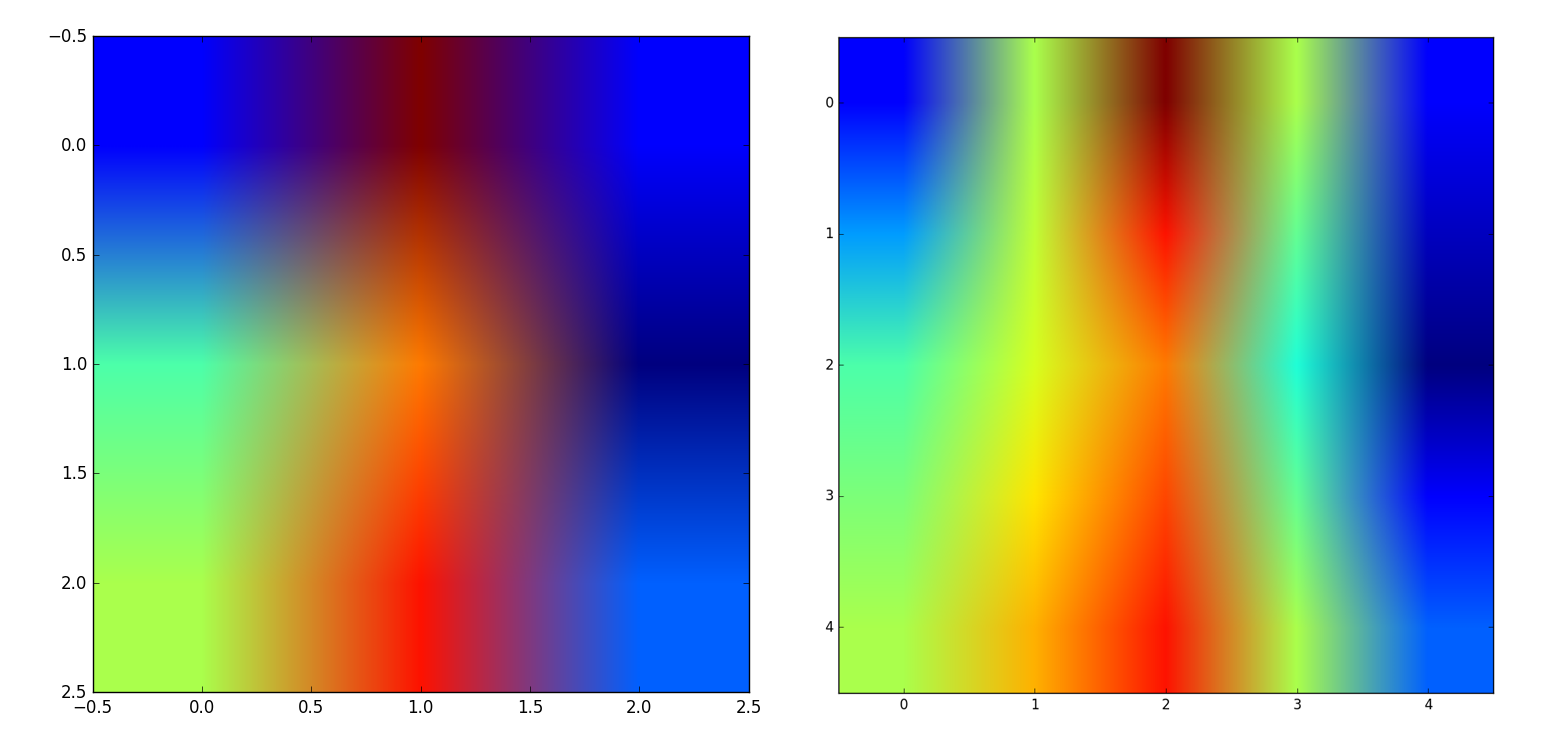## 拟合

### 使用numpy.polyfit拟合

#! python2
# coding: utf-8

import numpy
import pylab

def plot_polynomail_fit(y, *deg):
'''''
这个函数一次只拟合一组数据。但是可以对这一组数据同时拟合多条曲线并显示
y是一个list，存放的是需要拟合的数据
*deg是一个元组，长度不定,里面存放拟合的次数，可以对一组数据拟合出多条直线进行比较
'''

x = xrange(len(y))
COLOR = ['c','m','y','k','r','p','o','g','b']
temp = []
numOfLineToFit = len(deg) # 需要拟合的次数列表
for index,item in enumerate (deg):
param = numpy.polyfit(x,y,item) # 曲线的参数
equation = numpy.poly1d(param)   # 曲线方程
temp.extend(param[:])  # 提取曲线参数
print param
pylab.subplot(numOfLineToFit,1,index+1)
pylab.plot(x, equation(x),'%s--'%COLOR[index])
pylab.plot(x,y,'b--')

pylab.show()
return temp

if __name__ == '__main__':
y = [17, 19, 21, 28, 33, 38, 37, 37, 31, 23, 19, 18]
plot_polynomail_fit(y, 2, 3, 4)


3个拟合结果显示在下图中。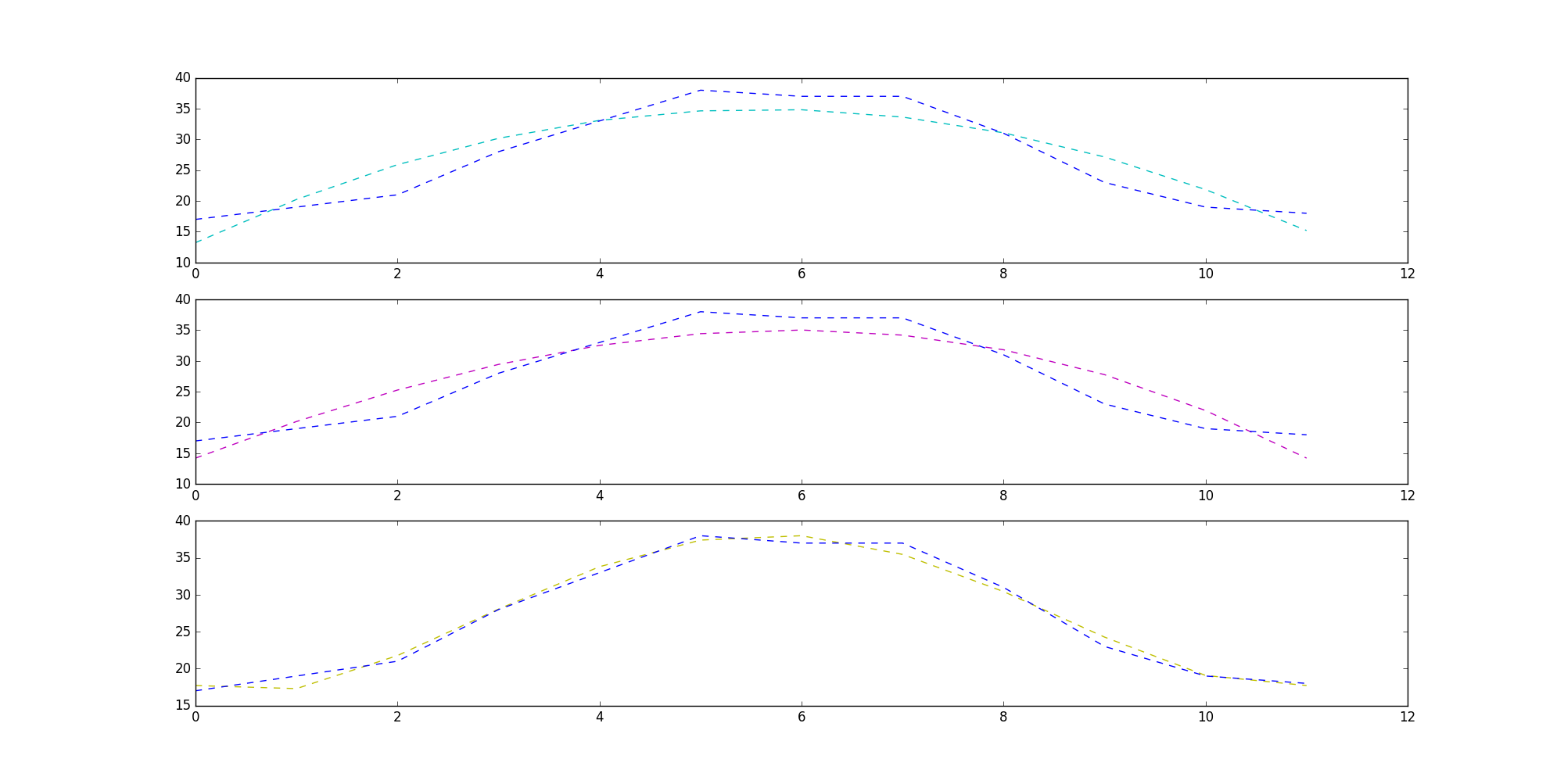### 使用scipy.optimize.optimize.curve_fit拟合

scipy提供的拟合，貌似需要先确定带参数的曲线方程，然后由scipy求解方程，返回曲线参数。我们还是以上面的一组数据为例使用scipy拟合曲线。

>>> import numpy as np
>>> import matplotlib.pyplot as plt
>>> from scipy import optimize
>>> x = np.arange(1,13,1)
>>> y = np.array([17,19,21,28,33,38,37,37,31,23,19,18 ])
>>> plt.plot(x, y)
[<matplotlib.lines.Line2D object at 0x04799D10>]
>>> plt.show()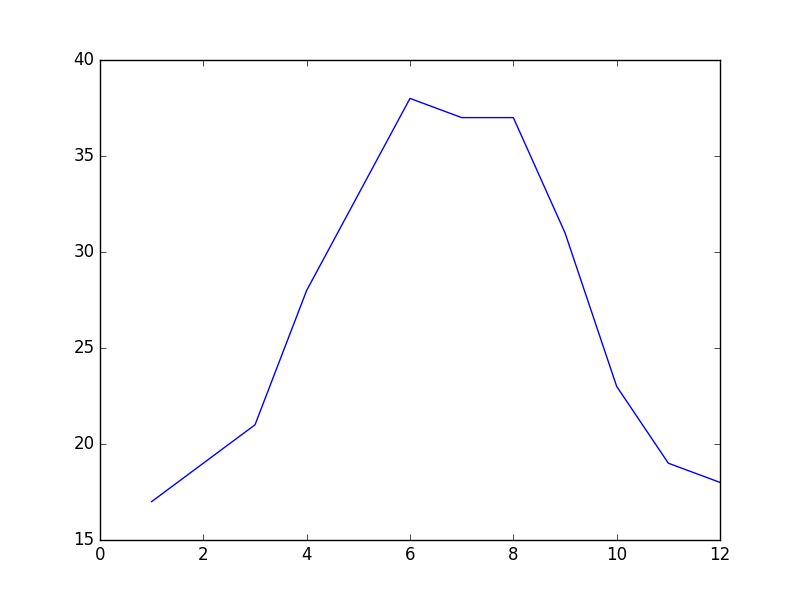>>> def fmax(x,a,b,c):
return a*np.sin(x*np.pi/6+b)+c

>>> fita, fitb = optimize.curve_fit(fmax, x, y, [1,1,1])
>>> print fita
[ 10.93254951  -1.9496096   26.75      ]
>>> xn = np.arange(1,13,0.1)
>>> plt.plot(x, y)
[<matplotlib.lines.Line2D object at 0x04B160B0>]
>>> plt.plot(xn, fmax(xn, fita,fita,fita))
[<matplotlib.lines.Line2D object at 0x04B16510>]
>>> plt.show()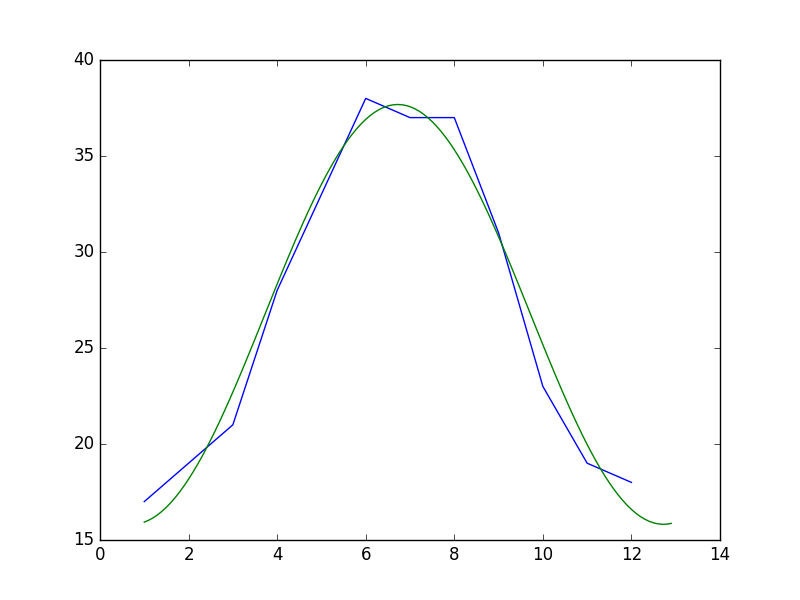## 求解非线性方程（组）

fsolve(func, x0)


func(x)是计算方程组误差的函数，它的参数x是一个矢量，表示方程组的各个未知数的一组可能解，func返回将x代入方程组之后得到的误差；x0为未知数矢量的初始值。

>>> from scipy.optimize import fsolve
>>> import numpy as np
>>> def f(A):
x = float(A)
return [np.sin(x) - np.cos(x) - 0.2]

>>> result = fsolve(f, )
array([ 0.92729522])
>>> print result
[0.92729522]
>>> print f(result)
[2.7977428707082197e-09]


$4x^2 - 2\sin(yz) = 0$
$5y + 3 = 0$
$yz - 1.5 = 0$

>>> from scipy.optimize import fsolve
>>> import numpy as np
>>> def f(A):
x = float(A)
y = float(A)
z = float(A)
return [4*x*x - 2*np.sin(y*z), 5*y + 3, y*z - 1.5]

>>> result = fsolve(f, [1, 1, 1])
>>> print result
[-0.70622057 -0.6        -2.5       ]
>>> print f(result)
[-9.1260332624187868e-14, 0.0, 5.329070518200751e-15]


## 数值积分

$x^2 + y^2 = 1$

$y = \sqrt{1 - x^2}$

>>> def half_circle(x):
return (1-x**2)**0.5


### 经典微分法

>>> N = 10000
>>> x = np.linspace(-1, 1, N)
>>> dx = 2.0/N
>>> y = half_circle(x)
>>> dx * np.sum(y[:-1] + y[1:]) # 面积的两倍
3.1412751679988937


### 使用定积分求解函数

>>> from scipy import integrate
>>> pi_half, err = integrate.quad(half_circle, -1, 1)
>>> pi_half*2
3.1415926535897984


## 图像处理

1. 载入Lena图像，并显示灰度图像
2. 应用中值滤波扫描信号的每一个数据点，并替换为相邻数据点的中值
3. 旋转图像
4. 应用Prewitt滤波器（基于图像强度的梯度计算）
>>> from scipy import misc
>>> from scipy import ndimage
>>> img = misc.lena().astype(np.float32)
>>> plt.subplot(221)
>>> plt.title('Original Image')
>>> plt.imshow(img, cmap=plt.cm.gray)
>>> plt.subplot(222)
>>> plt.title('Median Filter')
>>> filtered = ndimage.median_filter(img, size=(42,42))
>>> plt.imshow(filtered, cmap=plt.cm.gray)
>>> plt.subplot(223)
>>> plt.title('Rotated')
>>> rotated = ndimage.rotate(img, 90)
>>> plt.imshow(rotated, cmap=plt.cm.gray)
>>> plt.subplot(224)
>>> plt.title('Prewitt Filter')
>>> filtered = ndimage.prewitt(img)
>>> plt.imshow(filtered, cmap=plt.cm.gray)
>>> plt.show()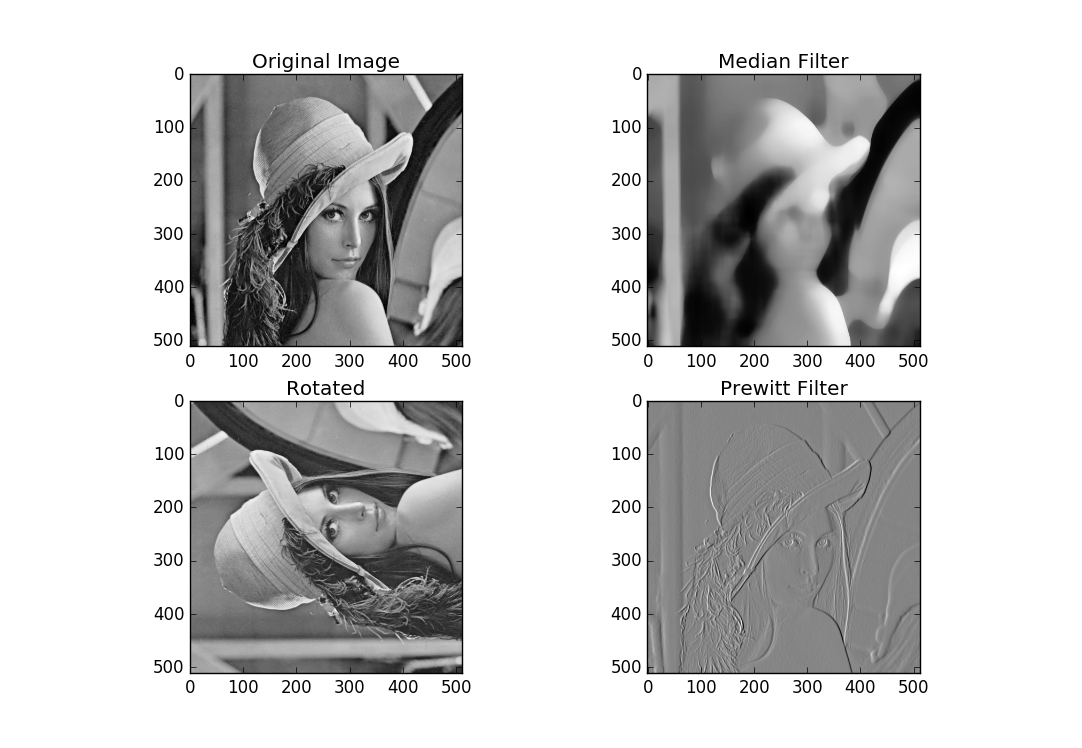# 后记